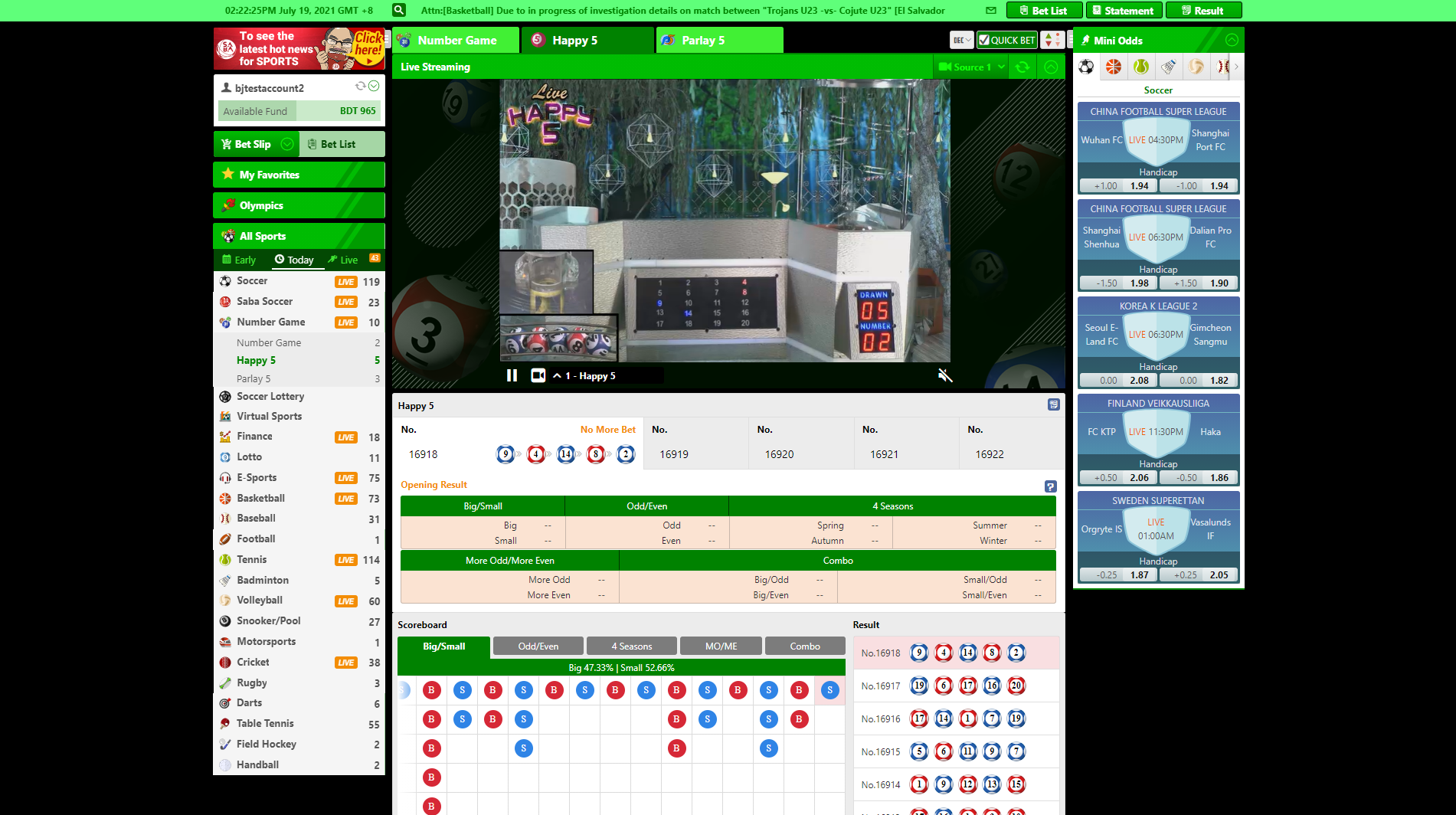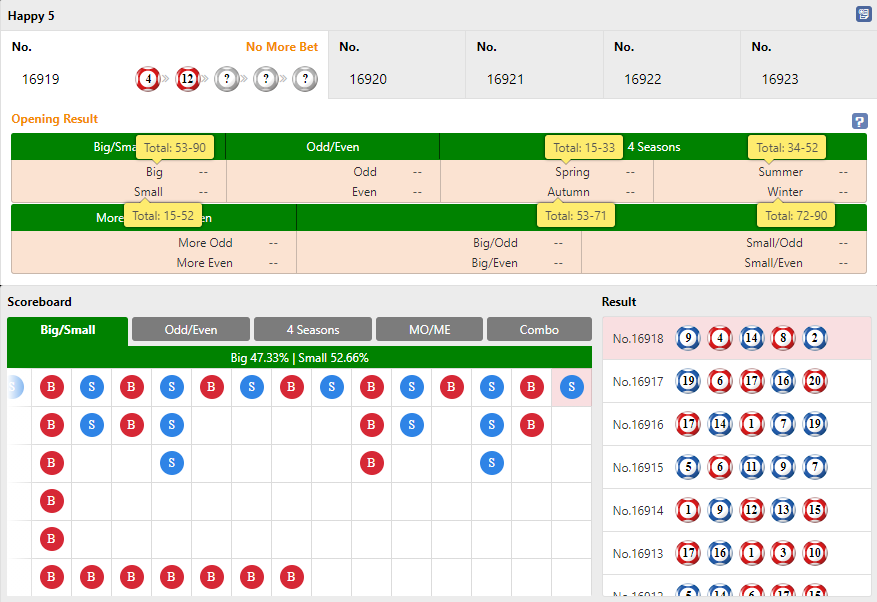What is a Happy 5?

Happy 5 is played by randomly drawing 5 balls from 20 numbered balls (01 to 20). The results are based on the combination of the 5 numbers drawn.

There are 5 bet types:

1. Big/Small

The total sum of the 5 numbered balls drawn is greater than or equal to 53 (Big); smaller than or equal to 52 (Small).

1. Odd/Even

From the total sum of the 5 numbered balls drawn, the last digit is an odd or an even number.

1. More Odd/More Even

From the 5 numbered balls drawn, if more than three of these numbers are odd numbers, bets on Odds win. If more than three of these numbers are even numbers, bets on Evens win.

1. 4 Seasons

Predict the total sum of the 5 numbered balls drawn will fall in a specific range name. There are 4 different range names to bet on: 15-33 (Spring), 34-52 (Summer), 53-71 (Autumn) and 72-90 (Winter).

1. Combo

For example, a BIG/ODD bet means you predict that the total sum of the 5 numbered balls drawn is greater than 53 and the last digit from the total sum is an odd number. Other combinations you can bet on are BIG/EVEN, SMALL/ODD and SMALL/EVEN.